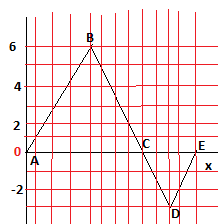# The force acting on a particle varies as in the figure below. Find the work done

The force acting on a particle varies as in the figure below. (The x axis is marked in increments of 0.500 m.) Find the work done by the force as the particle moves across the following distances.
a) from x = 0 m to x = 4.00 m
b) from x = 4.00 m to x = 6.00 m
c) from x = 0 m to x = 6.00 m J.